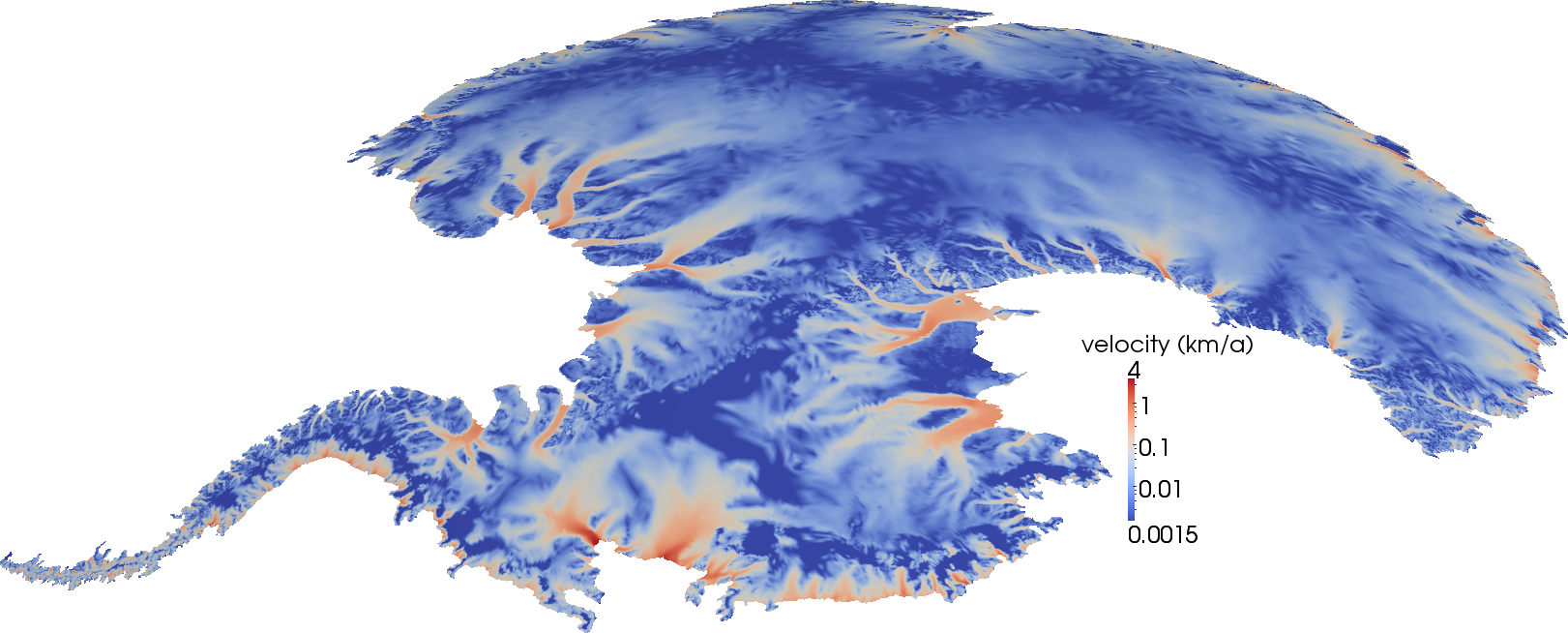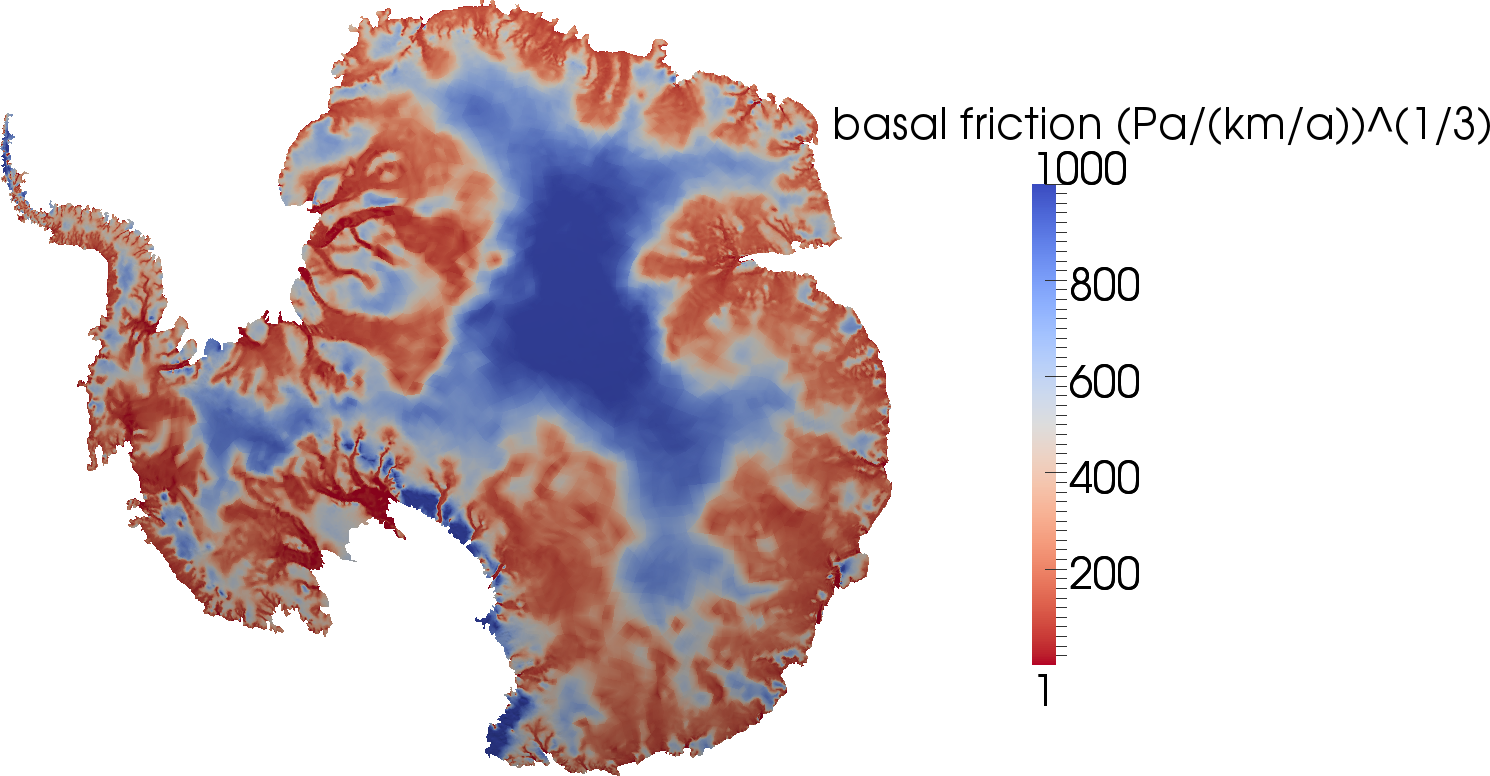### MATH 292 Special Topics: Computational and Variational Inverse ProblemsThis course provides an introduction to the numerical solution of inverse problems that are governed by systems of partial differential equations (PDEs). The focus of the course is on variational formulations, ill-posedness, regularization, variational discretization, and large-scale solution algorithms for inverse problems. Students will develop numerical implementations for model problems using a python-based high-level finite element toolkit (FEniCS). These implementations will allow us to study the influence of data noise, regularization, the observation operator, the choice of the parameter field, and the nature of the underlying PDE model on the identifiability of the model parameters, as well as facilitating experimentation with different solution algorithms. Examples will be drawn from different areas of science and engineering, including image processing, continuum mechanics, and geophysics. (The above figures show satellite observations of surface ice flow velocity used to solve an Antarctic ice sheet inverse problem (left) and the inferred basal friction parameter field (right).)

Prerequisites: Graduate standing or consent of instructors. Background in numerical linear algebra, partial differential equations, and nonlinear optimization is desirable. However, the required mathematical background will be covered when needed—albeit quickly.

Required work: About six assignments involving a mix of theory, implementation, and computational experiments and a final project.

Syllabus: (and more) coming soon! Stay tuned! If interested on this class and have questions, please do not hesitate to contact me.